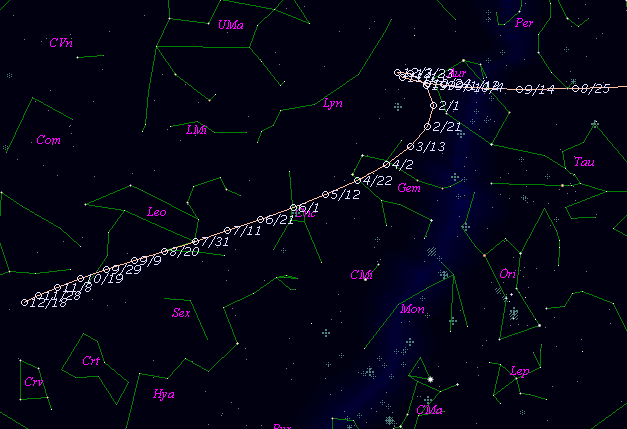\$B%m%t%!%7%eBh#1WB@1(B

93P/Lovas 1 (1998)\$B\$B50F;MWAG(B

```   The following improved orbital elements by Kenji Muraoka,
are from 117 observations 1980 to 1998, including 9 Planets,
Moon and 5 minor planets perturbations and non-gravitational
effect of style II.  The mean residual is +/- 0.70 arc seconds.

Epoch  =  1998 Nov.  3.0  TT       JDT = 2451120.5
T  =  1998 Oct. 14.14962       +/- 0.00182 (m.e.) TT
Peri. =   74.49216                +/- 0.00074
Node  =  340.01962                +/- 0.00011   (2000.0)
Incl. =   12.23659                +/- 0.00002
q  =    1.6917872              +/- 0.0000012 AU
e  =    0.6131189              +/- 0.0000010
a  =    4.3728868              +/- 0.0000027 AU
n  =    0.10778336             +/- 0.00000010
P  =    9.144                  +/- 0.0000084  years
A1  =   +0.396                  +/- 0.117
A2  =   -0.00349                +/- 0.00041
```\$B@1?^(B\$B8wEYJQ2=(B

```        m1 =  6.5 + 5 log\$B&\$(B + 30.0 log r  [ ,0]  (              \$B!A(B1998\$BG/(B10\$B7n(B14\$BF|(B)
m1 = 10.0 + 5 log\$B&\$(B + 15.0 log r  [0, ]  (1998\$BG/(B10\$B7n(B14\$BF|!A(B              )
```\$B50F;MWAG\$OB<2,7r<#;a\$N7W;;\$K\$h\$k\$b\$N\$G\$9!#(B \$B@1?^\$O(B StellaNavigator Ver.2.0 for Windows (\$B%"%9%H%m%"!<%D(B \$BJTCx(B / \$B%"%9%-!<=PHG6I4)(B) \$B\$G:n@.\$7\$?\$b\$N\$G\$9!#(B \$B8wEY%0%i%U\$O(BComet for Windows\$B\$G:n@.\$7\$?\$b\$N\$G\$9!#(B